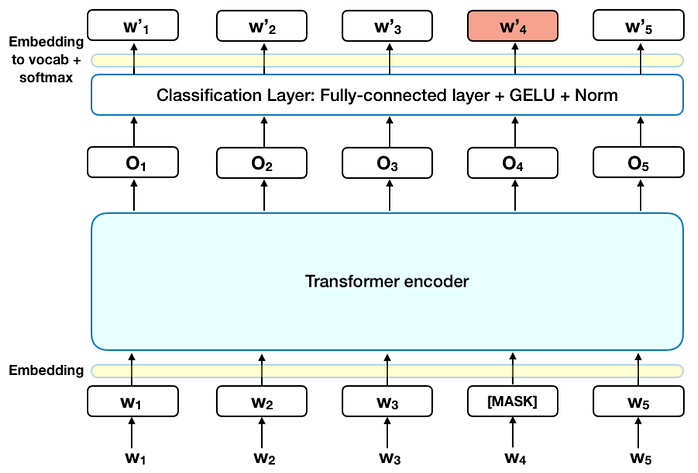# What does the outputlayer of BERT for masked language modelling look like?

In the tutorial BERT – State of the Art Language Model for NLP the masked language modeling pre-training steps are described as follows:

In technical terms, the prediction of the output words requires:

1. Adding a classification layer on top of the encoder output.

2.Multiplying the output vectors by the embedding matrix, transforming them into the vocabulary dimension.

3.Calculating the probability of each word in the vocabulary with softmax.

In the Figure below this process is visualized and also from the tutorial.

I am confused about what exactly is done. Does it mean that each output vector O is fed into a fully connected layer with embedding_size neurons and then multiplied by the embedding matrix from the input layer?

Update:

In the tutorial The Illustrated GPT-2 (Visualizing Transformer Language Models) I found an explanation for GPT-2 which seems to be similar to my question.

In the tutorial is said that each output vector is multiplied by the input embedding matrix to get the final output.

Does the same mechanic apply to BERT?